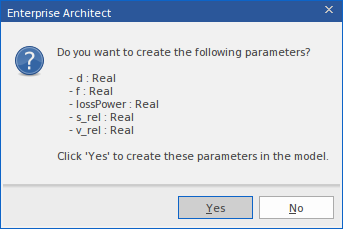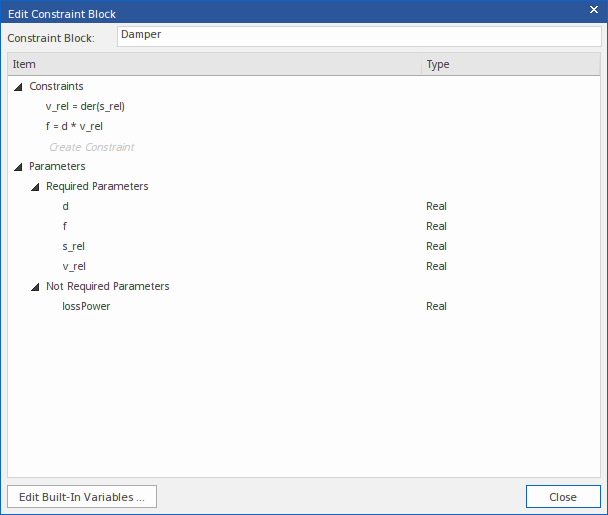﻿ Create a Constraint Block from Equations | Enterprise Architect User Guide

#### Please note : This help page is not for the latest version of Enterprise Architect. The latest help can be found here.

 Prev Next

# Create a Constraint Block from Equations

This feature is available from Enterprise Architect Release 14.1.

When developing an engineering solution, it is a common requirement to reflect factors determined by calculation using mathematical equations, such as Force = Mass x Acceleration (or f=m*a). The equation is represented by a Constraint, and the elements of the equation - in this case f, m, and a - are the parameters of the constraint.

You can model one or more calculated constraints as a SysML Constraint Block element using the 'Edit Constraint Block' dialog, through which you parse the constraints and extract the parameters from each of constraints. You can apply any equations that are appropriate to your model, whether they be international standard formulae or those you have derived yourself within the domain of your work.

## Access

 Context menu Right-click on a Constraint Block | Edit ConstraintBlock Other Diagram Toolbox, SysML Block Definition page | Drag a Constraint Block icon and drop it on a Block Definition diagram

## Parse Equations and create Parameters

Suppose we have a Constraint Block named 'Damper', containing these three equations as constraints:

• v_rel = der(s_rel)
• f = d * v_rel       ('d' is an incorrect symbol for 'Damping Coefficient'; this is deliberate, to allow correction in a later step)
• lossPower = f * v_rel

The three constraints are entered into the dialog (by overtyping the Create Constraint text) and from these constraints five parameters are automatically extracted.The '+' sign preceding each parameter indicates that it does not yet exist in the model. To create the parameter in the model:

1. Click on the .

The system displays a prompt to select whether or not to create the parameters.2. Click on the .

Alternatively, in the 'Edit Constraint Block' dialog, you can right-click on a new parameter and choose the 'Create Parameter' context menu option.  In this way, you can create a single parameter.

This image shows the resulting Constraint Block Damper.## Deleting Parameters

The 'Edit Constraint Block' dialog can also be used to remove a constraint and its associated parameters from a Block.

In our example, suppose we open the dialog and delete the constraint:

lossPower = f * v_rel

(Right-click on the constraint and select the 'Delete' option.)

Parameters unique to the constraint (in this case the 'lossPower' parameter) will be moved under the heading 'Not Required Parameters'.You can now:

• Right-click on the parameter and choose the 'Delete Parameter' context menu option, or
• Right-click on the 'Not Required Parameters' heading and choose the 'Delete All Not-Required Parameters' option

## Rename existing parameters

In our example, suppose we open the dialog and change the constraint:

f = d * v_rel

to

f = dampingCoefficient * v_rel

(Click on the constraint, click on the point in the constraint to begin editing, and overtype or delete the text. Then click off the constraint.)

These changes will occur:

• Parameter 'dampingCoefficient' is extracted and reported as a new parameter (it does not exist in the current model)
• Parameter 'd' is reported as 'Not Required'

We can delete 'd' and create 'dampingCoefficient', as explained earlier; however, there might be binding connectors connecting to parameter 'd' and all we want is to rename parameter 'd' to 'dampingCoefficient'.  Therefore, a better solution is to right-click on the 'Not Required' parameter 'd' and choose the menu option Rename 'd' to 'dampingCoefficient'.(For information on binding parameters, see the Bind Parameters of a ConstraintProperty Help topic.)

## Mathematical Functions

The equation parser supports the use of mathematical functions (such as der(s_rel), earlier in this topic) within your constraint equations.  When specifying a function, there should be no spaces between the function name and the opening parenthesis.  The function parameters will be extracted as new constraint parameters, but the function name will not.

## Built-In Variables

An equation could contain variables that you prefer not to extract as constraint parameters. For instance, the simulation environment OpenModelica provides a number of built-in variables, so where the model is to be simulated under OpenModelica you would not want to duplicate those variables as extracted parameters. You can identify the built-in variables to the 'Edit Constraint Block' functionality, so that they are not extracted from your constraint equations, thus avoiding potential conflicts.

To define a list of variables that should not be extracted as parameters, on the 'Edit Constraint Block' dialog click on the .Then enter or add to the comma-separated list of built-in variable names.

For example, OpenModelica defines 'time' as a built-in variable, so we would add 'time' to the list for the Constraint Blocks. Then when we enter the equation 'r = cos(time)' only the parameter 'r' will be extracted.## Composite Constraint Block

In the development of more complex and/or sequential calculated constraints, you can construct composite Constraint Blocks to break down and sequence the calculations.

In this example, the Constraint Block K does not itself define any constraints, but as a composite inherits them from its three component Constraint Blocks K1, K2 and K1TimesK2. Constraint Block K inherits the five parameters a, b, c, d and K.Rather than extract the value of K from one calculation, the system will first calculate K1, then K2, and finally the product of K1 and K2, giving the value of K.

This structure also makes it easier to modify the input of certain elements of the calculation without affecting the other elements.